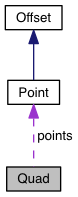PDG engine v0.9.5

4 point polygon in 2D system More...

Collaboration diagram for Quad:[legend]

## Public Member Functions

Quad (Point p1, Point p2, Point p3, Point p4)

Point centerPoint ()

boolean contains (Point p)

boolean equals (Quad q2)

Rect getBounds ()

moveDown (number delta)

moveLeft (number delta)

moveRight (number delta)

moveUp (number delta)

boolean notEquals (Quad q2)

rotate (number rotationRadians, Offset centerPtOffset=Point(0, 0))

Point[] points

## Detailed Description

4 point polygon in 2D system

Quad is a class that provides support for dealing with 4 point ploygons in 2 dimensional space The PDG coordinate space starts with the top left corner of the drawing port at Point(0,0) and the bottom left corner at Point( width, height );

## Constructor & Destructor Documentation

 Quad ( Rect r )
 Quad ( RotatedRect r )
 Quad ( Point p1, Point p2, Point p3, Point p4 )
 Quad ( Point[] p )

## Member Function Documentation

 Point centerPoint ( )
 boolean contains ( Point p )
 boolean equals ( Quad q2 )
 Rect getBounds ( )
 moveDown ( number delta )
 moveLeft ( number delta )
 moveRight ( number delta )
 moveUp ( number delta )
 boolean notEquals ( Quad q2 )
 rotate ( number rotationRadians, Offset centerPtOffset = `Point(0, 0)` )

## Member Data Documentation

 Point [] points